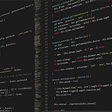# Codeforces 1270 G

Solution:

This is a very interesting problem.

note the special condition on a[i]

It is the same as 1≤i-a[i]≤N

If we let b[i]=i-a[i],then 1≤b[i]≤N

If sum{a[i]}=0, then sum{b[i]}=sum{i}

If we view i->b[i] as a directed edge, then we will observe this:

The solution set of indices form a cycle.

Why does cycle work?

Let S=cycle set of indices

Let T={b[x]|x in S}

We will see that T=S, and thus sum{T}=sum{S}

Do we always have a cycle?

YES! (By sense, I don’t have proof here)

So we just need to build our DAG and run Tarjan, output one cycle and we are done.

Aspiration:

I asked one of my friend who is really good at math for advice, here is his note:

The idea is to have a mapping from {i} to {b[i]} and then try to iterate and find a fixed set of points.

S0={1,…,N},S1=b(S0),..,Sn=b(Sn)

stop when Sn=Sn-1, Sn will be answer

The action of moving to fixed point is like BFS from vertices and then shrink to a cycle eventually. In fact, each time we remap from b[i] to b[b[i]], we are taking on edge one time. So this explains it.

Which is very interesting.

--

--

--

## More from Edward Lu

Love podcasts or audiobooks? Learn on the go with our new app.

## Sigstore Project Update — May 2021## Flask based domain name information checker## My Roller Coaster Journey in Learning Serverless## NEW! One Call API for essential weather data## 5 things that make learning how to code harder## Why You’re Never Too Old to Set New Goals? | Shellye## Business Update — I’m officially a Squarespace Circle Member · Stephanie Kabi